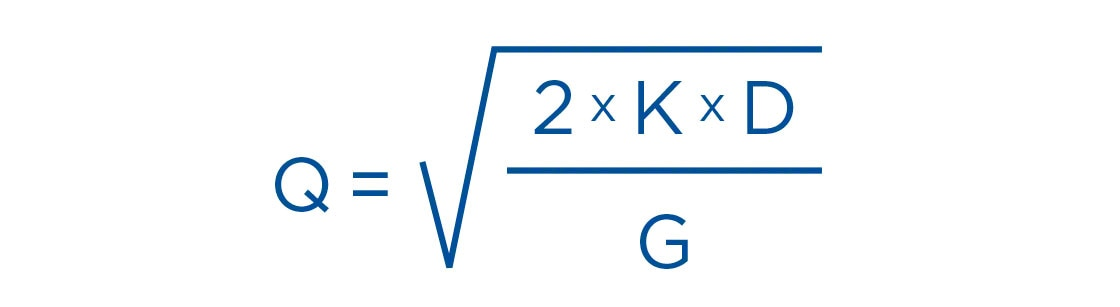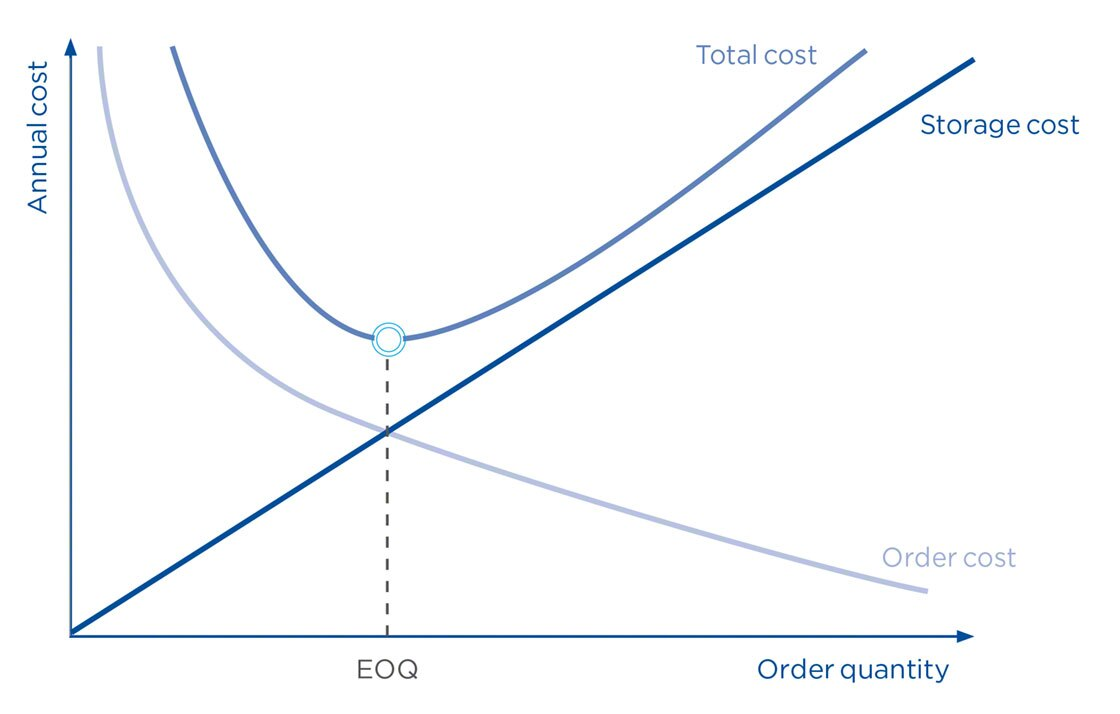# EOQ formula: the key to optimal stock management

25 March 2021

With a view to avoiding inventory issues, the EOQ (economic order quantity) formula or Wilson formula is a mathematical method for calculating how often to place an order with a supplier and how much to order, thus guaranteeing good stock management.

Although this formula is usually associated with the acquisition of raw materials and proper inventory management, the EOQ formula can actually be applied to any type of product.

## EOQ formula

Originally devised in 1913 by American engineer Ford Whitman Harris, it wasn’t until 1934 that consultant R.H. Wilson successfully developed the formula. But how is it calculated?

By taking a product’s demand, the cost of placing an order, and the storage cost, we can determine the optimal order volume. The mathematical formula is as follows:

• Q = optimal order quantity
• D = annual demand quantity of the raw material in question
• K = cost of placing the order
• G = cost of storing one unit in the warehouse for a specific amount of timeThe EOQ formula enables you to know when to place an order and in what quantity

## Applications and limitations

The objective of this mathematical model is to optimize the purchase volume of any required product, specifying when the order should be placed with the supplier and the exact amount of product.

With this data, we can also determine the reorder point (when to replenish your inventory), taking into account lead time and safety stock. Nevertheless, the EOQ formula does have certain constraints. This mathematical solution is only applicable to businesses that meet these conditions:

• The company has a constant demand for raw material throughout the year.
• The product to be procured maintains a fixed cost throughout the year, without major fluctuations in price.

If, for example, the cost of the raw material varies according to seasonality, the EOQ formula would be ineffective, as it doesn’t consider that variable.

Therefore, this method is only advisable for certain organizations in which demand and cost remain relatively stable over the course of the year.

## Advantages of the EOQ formula

For companies that meet both criteria, this mathematical method ensures the optimization of inventory management. Namely, this solution will afford you the following benefits:

• Minimize procurement and storage costs.
• Prevent excess stock while guaranteeing sufficient inventory levels for satisfying demand at all times.
• Know the appropriate quantities to request in each order.
• Avoid stockouts.The ideal reorder point is deduced by crossing variables such as order quantity and annual cost

### EOQ formula: a practical exercise

Let’s imagine there’s a company called Orch Kork that manufactures and distributes corks for wine producers in its area. To satisfy its annual production of 10,000 units, throughout the year, the business procures 2,000 lb of cork oak.

If each order costs \$200, including transportation expenses, and the cost of storing the product is under \$3,000 a year, what’s the ideal order amount?

In this case, Q (the optimal order quantity) would be solved from the square root of the formula 2*200*2000/3000, the result of which is 16.32.

Orch Kork should place annual orders of 16 lb in order to have the most appropriate number of pounds of cork oak to produce cork, thereby avoiding the excessive storage of raw material and potential stockouts. So, the company should place 125 annual orders of 16 lb to procure the 2,000 lb of cork oak.

## EOQ formula: minimizing stock costs (without seasonality)

In short, thanks to the implementation of the EOQ formula in stock management, companies can leverage order acquisition and, hence, reduce their overall storage and purchasing costs. It’s important to remember, though, that this formula is suitable when demand and prices are constant during the course of the year. For more complex situations, the model wouldn’t be useful; in these cases, the answer lies in a warehouse management system (WMS).

Missconfigured or missplaced portlet, no content found
Dynamic Content: false
Master Name: Banner-Software-Solutions
Template Key: